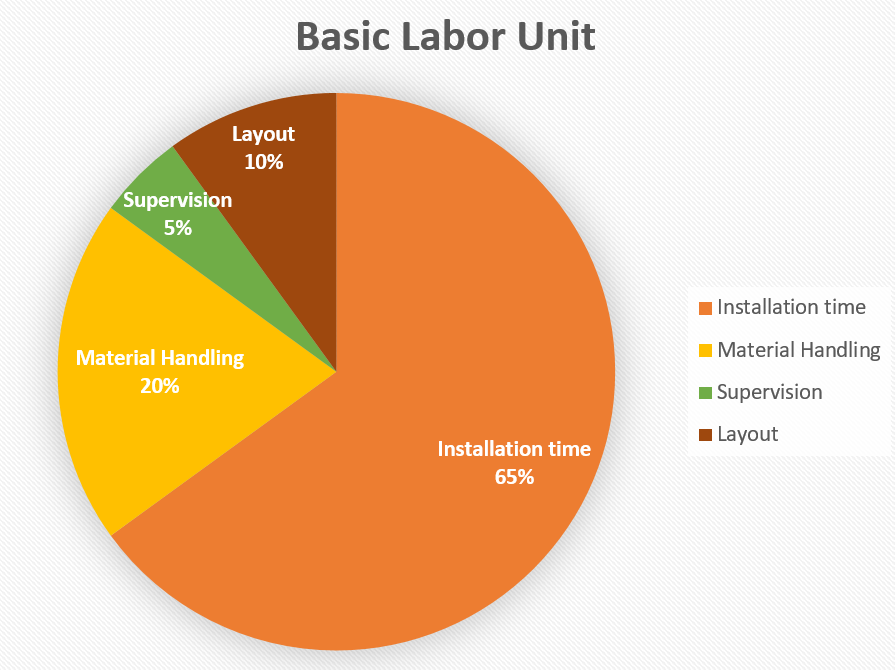Phone: 905-941-1611## Estimating Labor – Part 1

Understanding labor and the factors that impact labor costs is vitally important. Most of the risks in construction are labor risks. There are so many factors that can cause a project to lose monies.

Every estimator must know the ingredients that compose a labor unit. And he must know how to do the math to calculate his own labor units for specific tasks.

1st – The Labor Unit

The definition of a labor unit = a unit of time for the installation of material based on project conditions.

An estimator needs to understand the composition of a labor unit. A basic labor unit is 65% installation time and 35% for layout, material handling, clean up, and supervision.If you need to add a special labor task that is not in your estimating database, you can add a temporary or special item in your estimating program. So, I estimate how long it would take me to install the material and then I add 35% to the labor unit.

Example – If I thought that something would take 1 hour to install, 1.00 + .35 = 1.35 labor unit.

2nd – Estimating Math

An estimator must understand estimating math. To calculate a labor unit, you must know the following:

1. Number of men
2. Hours of each man
3. Total hours
4. Total output

Formula = 1 × 2 = 3 ÷ 4

2 men worked 8 hours = 16 man-hours ÷ 200 ft. of 2” GRC = 8.00 C

Labor Unit = Men × Hours Each = Total Hrs ÷ Total Output

Here are some examples:

 Material Installed Men Hours Total Hrs. Quantity Labor Unit E,C,M 3/4” EMT 1 4 4 100 ft. 4.00 C 4” PVC – in trench 3 8 24 200 ft. 12.00 C 4000 AMP Main Switchboard 4 8 32 1 32 E 300 KVA Transformer 3 5 15 1 15 E

In my next training tip, I will explain the difference between “Factoring Labor” and “Labor Factors.”

Do you know the difference?   Stay tuned for the next training tip.

Remember, estimating is expensive, poor estimating is costly, and quality estimating is profitable.